[Next]: Sharp interface model for eutectic tin/lead
 [Up]: Project descriptions
 [Previous]: On a nonlocal model of image
[Contents]   [Index]

## Upscaling of microscopically calculated characteristics to macroscopic state equations

Collaborator: U. Bandelow (FG 1), Th. Koprucki (FG 3)

Supported by: DFG: Priority Program ,,Analysis, Modellbildung und Simulation von Mehrskalenproblemen (Analysis, modeling and simulation of multiscale problems)

Description:

Eight-band kp-Hamiltonians  are detailed models for the calculation of quantum-confined states in semiconductor quantum-well structures, because they consistently include band mixing, spin-orbit interaction and strain effects. Quantities such as carrier densities or the optical response can be efficiently obtained in terms of the states (). To utilize such microscopically calculated data for device simulation, e.g., with drift-diffusion-type models , , a suitable upscaling to macroscopic state equations is required. We have demonstrated upscaling schemes for the carrier densities, the peak gain characteristics and the spontaneous radiative recombination rate calculated with WIAS-QW for an example quantum-well structure .

The investigations have been carried out for an InGaAsP-based single quantum-well structure which is designed for emission at 1.55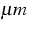consisting of a compressively strained quantum well of width dQW=7 nm sandwiched between tensile strained barriers.

As a first example for upscaling we consider the carrier densities. kp calculations  generically provide local carrier densities n(z) and p(z) depending on the Fermi levels Fe and Fh and the temperature T. Since the carriers localize very well in the quantum well  we can introduce an average carrier density per quantum well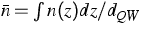as a natural quantity for upscaling. Thus we obtain from the microscopical model the relations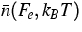and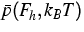for the averaged carrier densities as depicted in Figure 1. The upscaling to a macroscopic level can then be performed by fitting the calculated relationsandto macroscopic state equations such as the Fermi distribution used in drift-diffusion models. As shown in Figure 1 we observed that both quantities can be reasonably fitted to the relations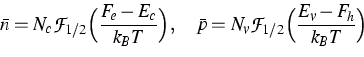(1)
by adjusting the effective band edges Ec and Ev and the band-edge densities of state Nc and Nv.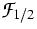is the Fermi integral of order 1/2. The fit for the valence bands is very close to the calculated curve for a wide range of densities and temperatures whereas for the conduction bands the macroscopic state equation yields a good approximation only for a certain range of parameters. Thus, an upscaling of band-structure information by the dependence of the average density per quantum well on the Fermi level is possible. This allows us to treat the quantum well as a classical material with microscopically defined band-edge densities of state Nc and Nv and net band edges Ec and Ev which differ from the band-structure parameters of the well material and cannot be estimated elsewhere.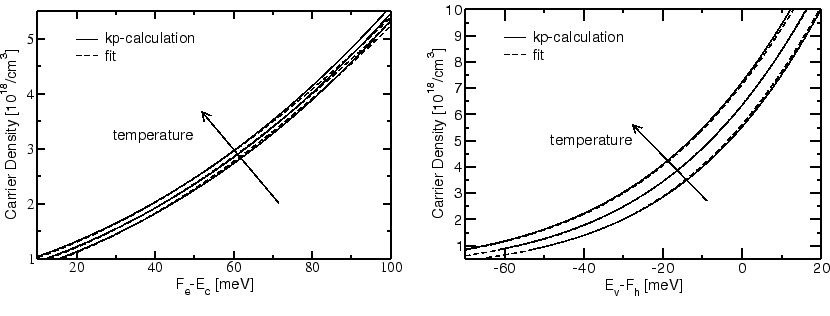Furthermore, the spectra of the optical material gain can be calculated in terms of the band-structure and transition matrix elements given by the wavefunctions , . This defines a function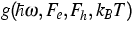depending on the transition energy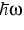, the Fermi levels Fe and Fh and the temperature T. Most lasers are designed to emit at the spectral gain maximum. Therefore the peak gain gp is an important quantity.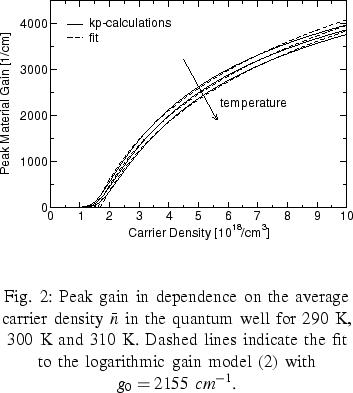As a second example we have calculated the peak gain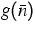(assuming charge neutrality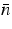=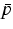) as a function of the average carrier density introduced above. The result is depicted in Figure 2. For sufficiently high carrier densities (above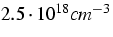) this peak gain characteristics can be upscaled to a usually used state equation given by the logarithmic gain model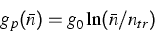(2)
as shown in Figure 2. However, for low densities this way of upscaling completely fails. The constant g0 appears to be nearly independent of the temperature whereas the transparency carrier density ntr should roughly linearly increase with the temperature.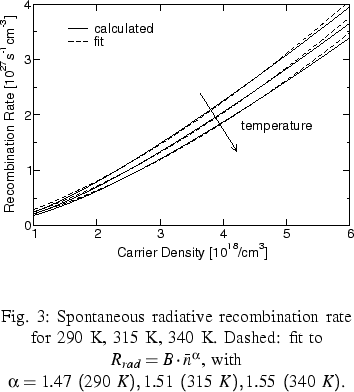As a third example we analyzed the upscaling of the spontaneous radiative recombination rate  which is a further important quantity for the analysis of semiconductor lasers, because it essentially determines the laser threshold. The spontaneous radiative recombination rate Rrad(Fe, Fh, T) obtained by the kp calculations depends on the Fermi levels Fe and Fh and the temperature. Again, for local charge neutrality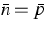we found out that this relation can be suitably upscaled to the power law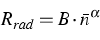as depicted in Figure 3. The exponent for the fit was approximately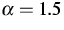, which differs from the commonly used models corresponding to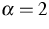.

References:

1. U. BANDELOW, H.-CHR. KAISER, TH. KOPRUCKI, J. REHBERG, Spectral properties of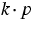Schrödinger operators in one space dimension, Numer. Funct. Anal. Optim., 21 (2000), pp. 379-409.
2., Modeling and simulation of strained quantum wells in semiconductor lasers, to appear in: Mathematics -- Key Technology for the Future II, K.-H. Hoffmann, W. Jäger, T. Lohmann, H. Schunck, eds., Springer, Berlin, Heidelberg.
3. U. BANDELOW, R. HÜNLICH, TH. KOPRUCKI, Simulation of static and dynamic properties of edge-emitting multi quantum well lasers, WIAS Preprint no. 799 , 2002.

 [Next]: Sharp interface model for eutectic tin/lead
 [Up]: Project descriptions
 [Previous]: On a nonlocal model of image
[Contents]   [Index]

LaTeX typesetting by I. Bremer
5/16/2003